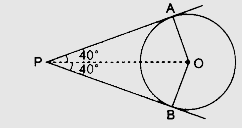# If tangents $PA$ and $PB$ from a point $P$ to a circle with centre $O$ are inclined to each other at angle of $80^o$, then $‚àÝPOA$ is equal to(a) $50^o$(b) $60^o$(c) $70^o$(d) $80^o$

Given:

Tangents $PA$ and $PB$ from a point $P$ to a circle with centre $O$ are inclined to each other at angle of $80^o$.

To do:

We have to find $∠POA$.

Solution:We know that,

The tangent to a circle is perpendicular to the radius through the point of contact.

The lengths of the tangents from an external point are equal.

This implies,

In $\triangle OAP$ and $\triangle OBP$,

$OA = OB$

$PA = PB$

$OP = OP$

Therefore, by SSS congruence,

$\triangle OAP \cong \triangle OBP$

This implies,

$\angle AOB + \angle APB = 180^o$

$\angle AOB + 80^o = 180^o$

$\angle AOB = 180^o - 80^o$

$\angle AOB = 100^o$

This implies,

$\angle POA=\frac{1}{2}\times100^o$

$=50^o$

Therefore, $∠POA$ is equal to $50^o$.